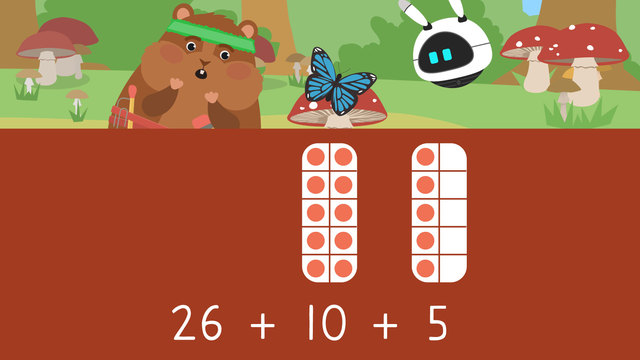# Using Make Ten to AddRating

Ø 5.0 / 2 ratings
The authorsTeam Digital
CCSS.MATH.CONTENT.OA.B.2

## Making a Ten Strategy – Understanding Ten Frames

The ability to use appropriate tools when performing addition is critical for developing a deep understanding of addition operations. A ten frame is one such tool.

A ten frame is a rectangle with ten equal spaces, divided into two rows of five. It is used to represent numbers less than or equal to ten. Since ten frames contain ten equal spaces, they allow children to develop numbers sense and exchange skills within the base 10 number system. The ten frame can be used when using the make a ten addition strategy.

## How to Use a Ten Frame

We use a ten frame by placing a single counter in each of the spaces to make the number. When adding, we can use ten frames to help us break up numbers and count on. A double-digit number can be broken up into the tens and ones. The tens place will fill up the ten frame.

For example, the number seventeen can be broken up into a ten and seven ones that can be shown on the ten frame## How to Use Make a Ten to Add

The ten frame can, therefore, be used to add larger numbers by breaking apart the smaller addend into tens and ones.

For example, if we are asked to add 38 and 15 we can do the following:

• Step 1: Create a number sentence → 38 + 15

• Step 2: Break apart the smaller addend and place it in the ten frame. The smaller addend is 15, therefore, 15 becomes 10 and 5• Step 3: Solve using the ten frame by adding ten and counting on. Therefore, the complete ten frame combines with 38 to give us 48. Then, we count on from there using the second ten frame to get 53.

### Ten Frames – Steps for Making Ten to Add

To use make a ten to add, we can follow these steps:

Step What to do
1. Create a number sentence.
2. Break apart the smaller addend.
3. Solve using the ten frame by
adding to the bigger number in
tens and then counting on.

## Making 10 to Add – Summary

Let’s repeat what we learned about ten frames and the strategy to make ten to add today.

Ten frames are useful tools in an addition strategy where the smaller addend can be broken apart into a ten and the smaller number represented on another ten frame. The ten frame can then be used to find the sum by counting in tens and then counting on. Because ten frames provide a visual representation of numbers, they allow children to make sense of ‘tens’ and ‘ones’

What is a ten frame?
Why are ten frames important?
What is number sense and how does it relate to ten frames?
Can ten frames be used to teach addition and subtraction?
Are ten frames relevant beyond kindergarten?
How can ten frames help with teaching larger numbers?
Can ten frames be used to teach number bonds?

## Using Make Ten to Add exercise

Would you like to apply the knowledge you’ve learned? You can review and practice it with the tasks for the video Using Make Ten to Add.
• ### How do you use make ten to add?

Hints

Remember, the first thing you need to do is understand what the problem is asking, and create an equation to show that in a math sentence.

Try adding 23 + 14 on your own. What steps did you follow?

Solution

For Mr. Squeaks to teach Imani, he needs to show them these steps in this order.

• ### Select the correct make tens equation.

Hints

You will break the number 23 (the smaller addend) into tens.

Think about the number 23. How many tens are in 20?

Solution

27 + 23 would be 27 + 10 + 10 + 3. We split the smaller addend (23) into tens (20), with three ones left.

• ### Match the pairs.

Hints

14 + 11 in make 10 is 14 + 10 + 1.

Remember, in make 10, we break the smaller addend into tens and ones.

Example: 17 + 12 = 17 + 10 + 2

Solution

When we use make 10, we break the smaller number into tens and ones, like these pairs.

• ### Show the steps.

Hints

First, we need to break the smaller addend into tens and ones.

For the first step, 12 would be broken into 10 + 2.

For the second step, add 18 + 10 to fill in the blank.

Solution

Mr. Squeaks can use make 10 to find the answer! He will start by breaking the smaller addend into tens ( 18 + 10 + 2). Next, he will add the ten to the larger addend (28 + 2). Finally, he will add on the ones (30). The answer is 30.

• ### How much in total?

Hints

Solution

By using make ten, we can add the numbers together.

31 + 10 + 7.

First, add the 31 + 10. 31 + 10 = 41.

Next, add the one place. 41 + 7 = 48.

Hints

Remember, break the smaller addend (16) into tens and ones.